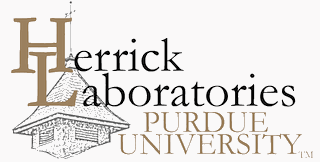## International Refrigeration and Air Conditioning Conference

2021

#### Keywords

numerical analysis, computational efficiency, thermophysical properties, phase equilibria, REFPROP

#### Abstract

Computationally efficient simulation of thermal systems is usually limited by evaluation of thermophysical properties. It is common (for instance in heat exchanger simulation) that more than 90% of the total runtime may be spent evaluating thermophysical properties. A key (and computationally expensive, especially for mixtures) part of that calculation is the determination of phase equilibrium. This work demonstrates that the use of Chebyshev expansions combined with extended precision calculations allows for a non-iterative numerical formulation that is more accurate than the iterative calculations with the full equation of state and is also much faster to evaluate. In essence, this approach makes phase equilibrium calculations of pure fluids from equations of state obsolete.

COinS